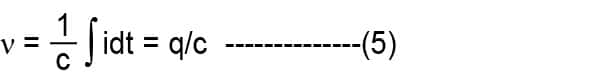# High-Frequency Issues In Electronics

K. Sita Rama Rao is scientist ‘G,’ R&QA, RCI, Hyderabad

21498

This article covers some important issues in design of high-frequency electronic circuits and commonly followed practices to mitigate them.

Electronic circuits behave very differently at high frequencies. This is mainly due to a change in the behaviour of passive components (resistors, inductors and capacitors) and parasitic effects on active components, PCB tracks and grounding patterns at high frequencies. The change of behaviour also causes electromagnetic interference (EMI) problems. This article discusses these effects and some commonly followed practices to mitigate them.## Ideal components

### Resistor

In time domain, the instantaneous voltage and current for an ideal resistor are related by:

v=iR ——-(1)

where R is the resistance and v the energy lost per unit charge when it flows from positive terminal to negative terminal. The energy lost is converted into heat by the resistor.

In frequency domain, phasors for sinusoidal voltages and currents are related by:

V=IR ———-(2)

Thus the voltage-current relationship is independent of frequency.

### Inductor (energy storage element)

In time domain, voltage-current relationship for an ideal inductor is:

v=Ldi/dt ———(3)

where L is the inductance and t the time.

Since v is the energy lost per unit charge, when charge flows from the positive terminal to the negative terminal, this energy is used for increasing current as per the above equation. The magnetic field inside the inductor coil is proportional to the current. Hence the energy is used in building up the magnetic field and stored in the magnetic field.

In frequency domain, phasors for sinusoidal voltages and currents are related by:

V=j(Lω)×I ——–(4)

where L is the inductance in Henry, ω the angular frequency of driving sinusoid voltage source in radians/second and j=√–1

Hence the voltage-current relationship for inductors varies with frequency.

### Capacitor (energy storage element)

In time domain, voltage and current for an ideal capacitor are related as:where q is the charge accumulated on the capacitor at time t, c the capacitance and t the time.

The charge accumulated on the capacitor establishes an electric field between plates of the capacitor and thus the capacitor stores energy in the form of electric field.

In frequency domain, phasors for sinusoidal voltages and currents are related by:where C is the capacitance in Farad,  the angular frequency of driving sinusoid voltage source in radians/second and j=√–1

Hence, the voltage-current relationship for capacitor varies with frequency.

## Behaviour of components at high frequencies

### Wires

At high frequencies, wires behave as inductors (opposing changes in the current) besides their natural low resistance value.

### Resistors

At high frequencies, resistors behave as series inductors (opposing changes in the current) and parallel capacitors (opposing changes in the voltage) besides their natural resistance.

### Inductors

At high frequencies, inductors behave as series resistors and parallel capacitors besides their natural inductance.

### Capacitors

At high frequencies, capacitors behave as series resistors and series inductors besides their natural capacitance.

Thus, simple voltage/current relationships for ideal components are no longer valid at high frequencies and suitable circuit analysis methods are to be used by replacing them with their high-frequency equivalent circuits.

### PCB tracks

PCB tracks behave like wires at low frequencies. This behaviour is no longer sustained at high frequencies. To understand this behaviour, you have to understand how a signal is transmitted from a source to the receiver and transmission line theory. Fig. 8 shows a source/driver delivering signal to a load/receiver through wires (shown by lines).